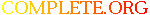Complete.Org: Mailing Lists: Archives: freeciv-dev: November 2001: [Freeciv-Dev] Re: [PATCH] base_real_map_distance (PR#1049)# [Freeciv-Dev] Re: [PATCH] base_real_map_distance (PR#1049)

[Top] [All Lists]

 To: freeciv-dev Subject: [Freeciv-Dev] Re: [PATCH] base_real_map_distance (PR#1049) From: Jason Dorje Short Date: Thu, 01 Nov 2001 14:46:51 -0500 Reply-to: jdorje@xxxxxxxxxxxx

```Raimar Falke wrote:
>
> On Thu, Nov 01, 2001 at 06:26:48AM -0800, jdorje@xxxxxxxxxxxxxxxxxxxxx wrote:
> > This patch introduces a new topology function,
> > base_real_map_distance().  It performs a similar function to
> > real_map_distance, but also finds a distance vector from the source to
> > the destination coordinate pair.  It also replaces xdist and ydist.
> >
> > A function like this is absolutely essential for iso-rectangular
> > topologies.  xdist() and ydist() will not work because you cannot find
> > the distance along one coordinate axis independently of the other.
> >
>
> > +  /* We assume base_real_map_distance gives us the vector with
> > +     the minimum squared distance.  Right now this is true. */
>
> > +  /* We assume base_real_map_distance gives us the vector with
> > +     the minimum map distance.  Right now this is true. */
>
> > +Topology function to find the minimum "real" distance between positions
> > +(x0, y0) and (x1, y1).  We find not only the distance but the vector
>
> The two comments indicate that the semantics and the description of
> base_real_map_distance could change. Why?

base_real_map_distance wouldn't change, but under a very strange
topology the difference vector it provides might not be the one that
sq_map_distance and map_distance need.  base_real_map_distance finds the
shortest "real map distance" from point A to point B, and provides the
vector that gives that shortest distance.  sq_map_distance and
map_distance are looking for something slightly different - but under a
topology that wraps in a linear-algebra type of way it will be correct.

In other words, base_real_map_distance is being used in a way it wasn't
strictly designed for.  A comment is necessary.

> >  {
> >    int diff_x, diff_y, dx, dy, scalar_product;
> >
> > -  if (dest_x > src_x) {
> > -    diff_x = dest_x - src_x < map.xsize / 2 ? 1 : -1;
> > -  } else if (dest_x < src_x) {
> > -    diff_x = src_x - dest_x < map.xsize / 2 ? -1 : 1;
> > -  } else {                   /* dest_x == src_x */
> > -    diff_x = 0;
> > -  }
> > -  if (dest_y != src_y)
> > -    diff_y = dest_y > src_y ? 1 : -1;
> > -  else
> > -    diff_y = 0;
> > +  base_real_map_distance(&diff_x, &diff_y, src_x, src_y, dest_x, dest_y);
>
> This looks wrong. Value range of old diff_[xy] was {-1,0,1}.

Yes, but for the scalar product computed below it won't matter:

scalar_product = diff_x * dx + diff_y * dy;

then realized it wasn't needed and dropped it.

straightest_direction actually has its behavior changed by this patch,
as the old code had a range {-1, 1} instead of {-1, 0, 1}.  Now
straightest_direction will actually point straight if you're on a line -
not that this is particularly useful, since the only time it is used is
for building roads (and maybe not for long even there).  I just figured
it was time to clean this one up, even if it does get dropped later on.

jason

```